# Scouts

Three scouts went on a three day trip. The second day went 4 km more than the first day. The third day went two times less than the first day. They went along 54 km. How many kilometers went every day together?

Result

a =  20 km
b =  24 km
c =  10 km

#### Solution:

b = 4+a
c = a/2
a+b+c = 54

a-b = -4
a-2c = 0
a+b+c = 54

a = 20
b = 24
c = 10

Calculated by our linear equations calculator.

Leave us a comment of example and its solution (i.e. if it is still somewhat unclear...):

Showing 0 comments:Be the first to comment!#### To solve this example are needed these knowledge from mathematics:

Do you have a linear equation or system of equations and looking for its solution? Or do you have quadratic equation?

## Next similar examples:

1. Two carsTwo cars started against each other at the same time to journey long 293 km. First car went 41 km/h and second 41 km/h. What distance will be between this cars 20 minutes before meet?
2. The cyclist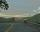The cyclist went from village to town. First half of journey went at 20 km/h. The second half of the journey, which mostly fell, went at 39 km/h. All journey took 88 minutes. Calculate the distance from the village to the town.
3. Pedestrian up-down hill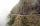Pedestrian goes for a walk first at plane at 4 km/h, then uphill 3 km/h. Then it is in the middle of the route, turns back and goes downhill at speed 6 km/h. Total walk was 6 hours. How many kilometers went pedestrian?
4. Bus vs. trainBus started from point A 10 minutes before the train started from the same place. The bus went an average speed of 49 km/h, train 77 km/h. To point B train and bus arrived simultaneously. Calculate time of train journey, if train and bus travelled the.
5. Motion2Cyclist started out of town at 19 km/h. After 0.7 hours car started behind him in the same direction and caught up with him for 23 minutes. How fast and how long went car from the city to caught cyclist?
6. Car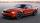Car goes from point A to point B at speed 86 km/h and back 53 km/h. If they goes there and back at speed 67 km/h trip would take 10 minutes shorter. What is distance between points A and B?
7. Trolleybus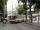Trolleybus line No. 206 measured 24 km. If the trolleybus goes faster by 5 km/h, the way there and back would is shorter by 33 minutes. Calculate the trolleybus speed and how much time it takes a return trip.
8. Tourists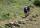From the cottage started first group of tourists at 10:00 AM at speed 4 km/h. The second started after them 47 minutes later at speed 6 km/h. How long and how many kilometers from the cottage will catch the first group?
9. MovementFrom the crossing of two perpendicular roads started two cyclists (each at different road). One runs at average speed 28 km/h, the second at average speed 24 km/h. Determine the distance between them after 45 minutes cycling.
10. Motion problemFrom Levíc to Košíc go car at speed 81 km/h. From Košíc to Levíc go another car at speed 69 km/h. How many minutes before the meeting will be cars 27 km away?
11. Cyclist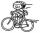Cyclist goes uphill 10 km for 50 minutes and downhill minutes for 29 minutes, both applied to the pedals same force. How long he pass 10 km by plane?
12. Reconstruction of the corridorCalculate how many minutes will be reduced to travel 187 km long railway corridor, where the maximum speed increases from 120 km/h to 160 km/h. Calculate how many minutes will shorten travel time, if we consider that the train must stop at 6 stations, eac
13. Car range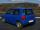Calculate the maximum range of car, if you can spend 10 euros, price of diesel is 1.55 Eur/l and car consumption is 3 l/100 km.
14. Train and car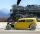The train and the car started at a constant speed to journey. When the train travels 87 km, the car travels 97 km. How many km travels the train when the car travels 87 km?
15. Rotation of the EarthCalculate the circumferential speed of the Earth's surface at a latitude of 61°​​. Consider a globe with a radius of 6378 km.
16. Speed of Slovakian trainsRudolf decided to take the train from the station 'Trnava' to 'Zemianske Kostoľany'. In the train timetables found train R 725 Remata : km0Bratislava hl.st.12:574Bratislava-Vinohrady13:0113:0219Pezinok13:1213:1346Trnava13:3013:3263Leopoldov13:4514:0168H
17. Car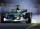Car is going 95 km per hour. How long distance goes in 1 minute?